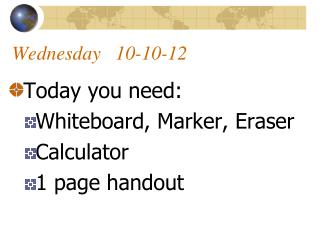DownloadDownload PresentationWednesday 10-10-12

# Wednesday 10-10-12

Télécharger la présentation## Wednesday 10-10-12

- - - - - - - - - - - - - - - - - - - - - - - - - - - E N D - - - - - - - - - - - - - - - - - - - - - - - - - - -
##### Presentation Transcript

1. Wednesday 10-10-12 • Today you need: • Whiteboard, Marker, Eraser • Calculator • 1 page handout

2. Warm-upNeed a White Board 1. Graph the following equation:

3. Warm-upNeed a White Board 1. Graph the following equation:

4. Linear Regression Section 2-6 Pages 95-100

5. Objectives • I can use Linear Regression with a calculator to find linear prediction Equations • I can find the correlation co-efficient “r” for the data

6. Correlation Co-efficient • The correlation co-efficient “r” tells how linear the data is. • Values of 1 or –1 indicate perfect linear lines, either positive or negative • Values closer to zero mean the data has no linear relationship • Small whiteboard number line with r=1 and r=-1

7. 1.0 .85 Sample “r Values -.57 .17

8. Plotting Data • When the data you plot forms a near linear relationship, then we can use a linear equation to approximate the graph. • We use what’s called a Best-Fit Line. This line is drawn to be as close to the data points as possible, but may not touch them all.

9. y-axis 45 40 35 30 25 20 15 10 5 x-axis 0 1 2 3 4 5 6 7 8 9 10

10. Using the Calculator (Linear Regression) • The calculator is a great resource to give us a prediction equation. • It is more accurate than doing the equation Manually • We will enter the data into the STAT mode of the calculator

11. Turn Diagnostics On. 2nd catalog, arrow to Diagnostic on, enter, enter Linear Regressions on the calculator: (you should clear the calculator before beginning) 2nd, +, 7, 1, 2 #1.

12. Linear Regression • Finding the equation of your “Best Fit Line” • STAT, then EDIT • Enter X-Values in L1, Y-Values in L2 • STAT, then CALC • Choose (4) LIN REG

13. y-axis 45 40 35 30 25 20 15 10 5 x-axis 0 1 2 3 4 5 6 7 8 9 10

14. The table below shows the years of experience for eight technicians at Lewis Techomatic and the hourly rate of pay each technician earns.

15. Prediction Equations • y = 1.234x + 5.574 • Remember: • x = Experience in Years • y = Pay rate in dollars • We can use this to predict other values

16. Predictions: 25 years experience

17. Predictions: \$32.72 per hour

18. When Dealing with Years • Must modify years starting at “0” • If you don’t you get a really negative y-intercept value that won’t match the graph • Example on next slide

19. If the Independent variable is Years and these are your values 1901 1903 1905 1910 1913 1920 Then these are the values we will actually enter for L1 0 2 4 9 12 19 Inputting Years

20. Homework • Linear Regression Ws